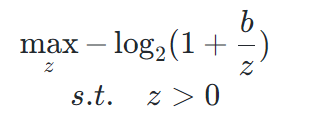# How to express the log function using cvxThere are a couple of formulations in Minimize log(1+1/x) where 0<x<inf . Use z/b in place of x, and divide everything by log(2) to make it log2 instead of log. You can get the same argmax without dividing by log(2).

The problem as stated will be considered as ill-posed, because, assuming b > 0, the optimal solution is z = \infty, which the solvers CVX call will never get to.